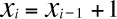## Chapter 6. Cellular Automata

A cellular automaton is a model of a world with very simple physics. “Cellular” means that the space is divided into discrete chunks, called cells. An “automaton” is a machine that performs computations—it could be a real machine, but more often the “machine” is a mathematical abstraction or a computer simulation.

Automata are governed by rules that determine how the system evolves in time. Time is divided into discrete steps, and the rules specify how to compute the state of the world during the next time step based on the current state.

As a trivial example, consider a cellular automaton (CA) with a single cell. The state of the cell is an integer represented by the variable xi, where the subscript i indicates that xi is the state of the system during time step i. As an initial condition,.

Now all we need is a rule. Arbitrarily, I’ll pick, which says that after each time step, the state of the CA gets incremented by 1. So far, we have a simple CA that performs a simple calculation: it counts.

But this CA is atypical; normally the number of possible states is finite. To bring it into line, I’ll choose the smallest interesting number of states, two, and another simple rule, , where is the remainder (or modulus) operator.

This CA performs a simple calculation: ...

Get Think Complexity now with the O’Reilly learning platform.

O’Reilly members experience live online training, plus books, videos, and digital content from nearly 200 publishers.